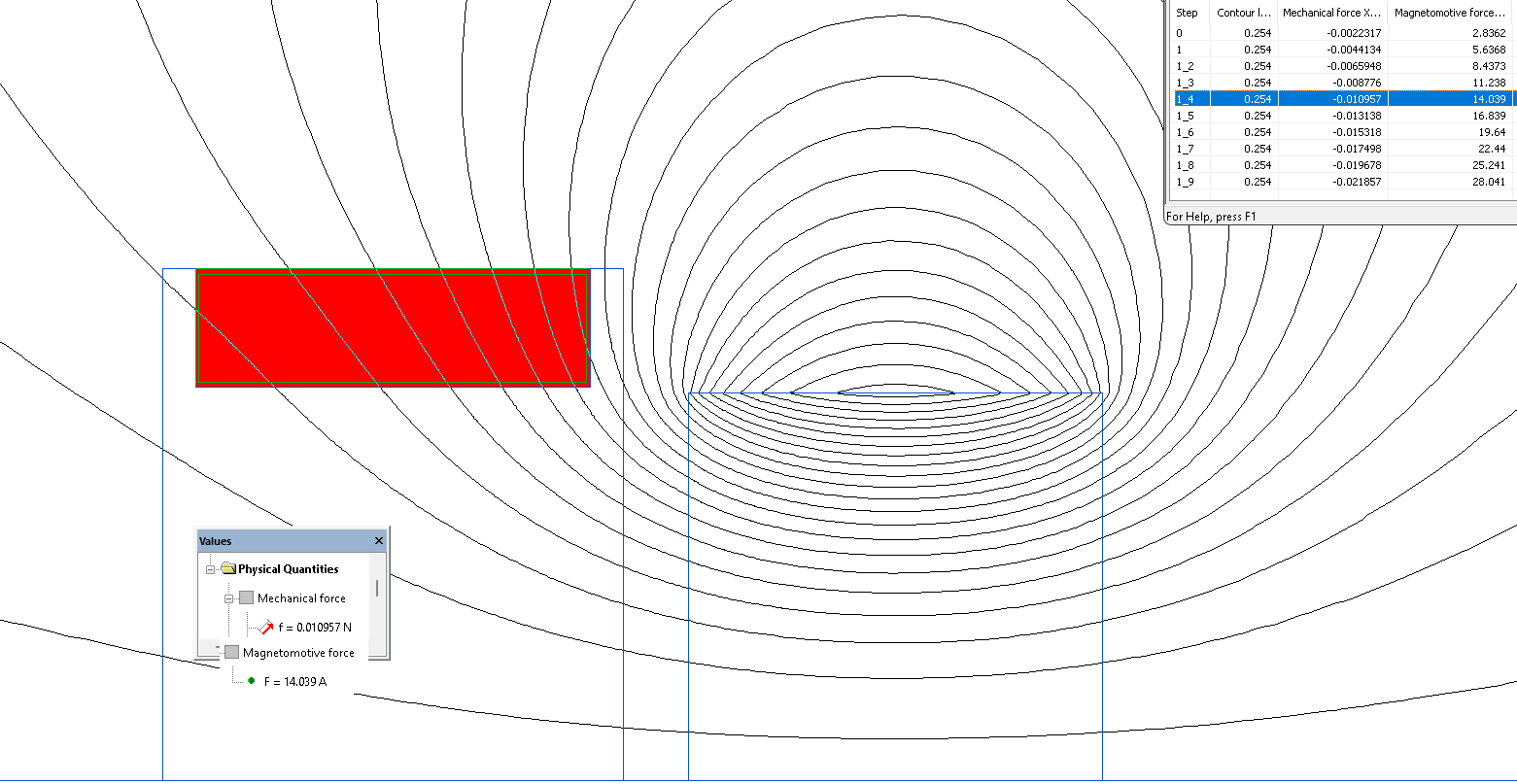QuickField

A new approach to field modelling

Main >> Applications >> Sample problems >> Permanent magnet force

# Permanent magnet force

QuickField simulation example

This is a simulation example from Compumag TEAM Workshop Problem 23*.

Problem Type
Axisymmetric problem of magnetostatics

Geometry

Given
Permanent magnet Sm-Co coercive force Hc = 720 kA/m, remanence Br = 1.06 T;
Coil number of turns 280, current I = 10.. 100 mA
Distance between the coil and the permanent magnet δ=0.254 mm.

Calculate the axial force between the permanent magnet and the coil and compare the results with the reference values.

Solution
The coil has a lot of turns and is wound with an insulated wire, so the current distribution across the coil cross-section is considered to be homogeneous. The coil is modelled as a solid block with the total ampere-turns specified.
LabelMover parametric tool is used to generate a series of problems and calculate the force.

Result
The force value at I = 50 mA is 0.01096 N, which is equal to the gravity force acting on a weight of 1.117 g (1.5% less than the reference value of 1.132 g).Reference
*Compumag TEAM Workshop